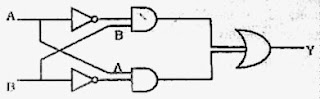## Sunday, February 21, 2010

### Two EAMCET (Medical) 2009 Questions on Photons and Electrons

Today we will discuss two multiple choice questions from modern physics. These questions are from the section ‘electrons and photons’ and they were included in the EAMCET (Medical) 2009 question paper:

(1) In Millikan’s oil drop experiment, a charged oil drop of mass 3.2×10–14 kg is held stationary between two parallel plates 6 mm apart, by applying a potential difference of 1200 V between them. How many electrons does the oil drop carry?

(1) 7

(2) 8

(3) 9

(4) 10

The electric field ‘E’ between the plates is V/d where V is the potential difference and d is the separation between the plates.

Therefore E = 1200/(6×10–3) = 2×105 volt per metre.

The electric force on the drop is Eq where ‘q’ is the charge on the drop. Since the drop is stationary, the electric force balances the weight mg of the drop.

Therefore Eq = mg so that

q = mg/E = (3.2×10–14×10)/( 2×105) = 1.6×10–18 coulomb.

Since the electronic charge is 1.6×10–19 coulomb, the number of excess electrons carried by the drop is (1.6×10–18) /(1.6×10–19) = 10.

(2) Electrons accelerated by a potential of ‘V’ volts strike a target material to produce ‘continuous X-rays’. Ratio between the de Broglie wave length of the electrons striking the target and the shortest wave length of the ‘continuous X-rays’ emitted is

(1) h/√(2Vem)

(2) (1/c) √(2m/Ve)

(3) (1/c) √(Ve/2m)

(4) he/√(Ve/2m)

The de Broglie wave length λ of the electron is given by

λ = h/p where h is Planck’s constant and p is the momentum of the electron.

The shortest wave length λmin of the ‘continuous X-rays’ produced by the striking electrons is given by

hc/λmin = Ve where c is the speed of light in free space and e is the electronic charge.

[Note that we have equated the entire energy of the electron to the energy of the X-ray photon for obtaining minimum wave length (or, the maximum frequency νmax):

eV =max = hc/λmin].

Therefore, λmin = hc/Ve.

The ratio of wave lengths required in the problem is

λ /λmin = (h/p)/(hc/Ve ) = Ve/pc………..(i)

The kinetic energy of the electron is eV and the momentum p of the electron is related to its kinetic energy as

eV = p2/2m where m is the mass of the electron.

Therefore, p = √(2mVe)

Substituting this in Eq (i), we obtain

λ /λmin = (1/c) √(Ve/2m)

## Wednesday, February 03, 2010

### Kerala Engineering and Medical Entrance Examination (KEAM) 2009 Questions on Digital Circuits

The following question appeared in Kerala Engineering Entrance 2009 Examination question paper:

The truth table for the following logic circuit isThe top AND gate gets inputs (NOT A) and B. The bottom AND gate gets inputs A and (NOT B). The outputs of the two AND gates are respectively [(NOT A) AND B] and [A AND (NOT B)]. These are the inputs for the final OR gate. The output of the final OR gate is [(NOT A) AND B] OR [A AND (NOT B)]. This is the Boolean expression for the XOR gate (exclusive OR gate, also termed EXOR gate). The truth table for the XOR gate is given in option (A).

Even if you don’t know the XOR gate and its truth table, you can substitute values 0 and 1 for the inputs A and B and find the output in each trial. You can easily arrive at the answer given in option (A).

The following question appeared in Kerala Medical Entrance 2009 Examination question paper:

If the two inputs of a NAND gate are shorted, the gate is equivalent to

(a) XOR

(b) OR

(c) NOR

(d) NOT

(e) AND

If both inputs of a two input NAND gate are shorted, both inputs get the same signal. Because of the NOT operation at the output side of the NAND, the output is the complement of the input. The circuit therefore behaves as a NOT gate [Option (d)].